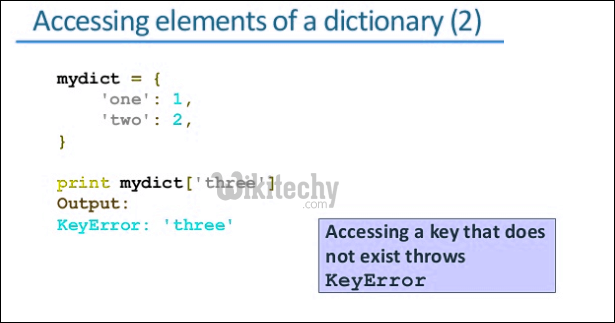# python tutorial - Dictionary Python | Dictionary Methods in Python | cmp(), len(), items()… - learn python - python programmingLearn Python - Python tutorial - dictionary python - Python examples - Python programs

1. str(dic) :- This method is used to return the string, denoting all the dictionary keys with their values.2. items() :- This method is used to return the list with all dictionary keys with values.

python - Sample - python code :

python tutorial - Output :

```The constituents of dictionary as string are :
{'Name': 'Nandini', 'Age': 19}
The constituents of dictionary as list are :
dict_items([('Name', 'Nandini'), ('Age', 19)])
```

3. len() :- It returns the count of key entities of the dictionary elements.4. type() :- This function returns the data type of the argument.

python - Sample - python code :

python tutorial - Output :

```The size of dic is : 3
The data type of dic is :
The data type of li is :
```

5. copy() :- This function creates the shallow copy of the dictionary into other dictionary.

6. clear() :- This function is used to clear the dictionary contents.

python - Sample - python code :

python tutorial - Output :

```The new copied dictionary is :
dict_items([('Age', 19), ('Name', 'Nandini')])
The contents of deleted dictionary is : dict_items([])
```

Wikitechy tutorial site provides you all the learn python , python course for beginners , learn python online interactive , how to learn python coding , best online python training , python online course certification free , python training for beginners , online python course free , learn python online free course